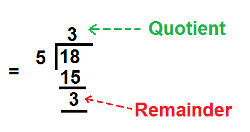Arithmetic
Arithmetic Progression
Associative Property
Averages
Brackets
Closure Property
Commutative Property
Conversion of Measurement Units
Cube Root
Decimal
Divisibility Principles
Equality
Exponents
Factors
Fractions
Fundamental Operations
H.C.F / G.C.D
Integers
L.C.M
Multiples
Multiplicative Identity
Multiplicative Inverse
Numbers
Percentages
Profit and Loss
Ratio and Proportion
Simple Interest
Square Root
Unitary Method
Algebra
Cartesian System
Order Relation
Polynomials
Probability
Standard Identities & their applications
Transpose
Geometry
Basic Geometrical Terms
Circle
Curves
Angles
Define Line, Line Segment and Rays
Non-Collinear Points
Parallelogram
Rectangle
Rhombus
Square
Three dimensional object
Trapezium
Triangle
Trigonometry
Trigonometry Ratios
Data-Handling
Arithmetic Mean
Frequency Distribution Table
Graphs
Median
Mode
Range

Videos
Solved Problems
Home >> Decimal >> Decimal to Mixed Fraction >>

## Convert Decimal to Mixed Fraction

 Decimal Point Place Value of digits in decimals Expanded Form of Decimal Read Decimals Decimal Places Decimal to Fraction Decimal to Mixed Fraction Decimal to Lowest Fractional form Decimal into Percentage Like Decimals Unlike Decimals Difference Like & Unlike Decimals Comparing or Ordering Decimals Ascending Order of Decimals Descending Order of Decimals Multiplication of Decimals Division of Decimals

Before you understand; How to convert Decimal to Mixed fraction, You must know:

What are Decimal Places ?
What is Decimal Point ?
What are Fractions ?
What are Mixed Fractions ?
How to Calculate H.C.F. ?

### Steps to convert Decimal to Mixed fraction

• Step 1: Numerator of fraction is equal to decimal (without the decimal point)
• Step 2: Find number of Decimal Place in the given decimal
• Step 3: Denominator of fraction is equal to 1 followed by as many number of zero as there are decimal place.

After Step 3, we will get a fraction, which will be further converted into lowest form, through following step:
• Step 4: Find HCF of numerator and denominator of fraction
• Step 5: Divide both numerator and denominator by HCF and we get fraction in lowest form

After Step 5, we will get a lowest fraction, which will be further converted into mixed fraction, through following steps:
• Step 6: Divide Numerator by denominator.
• Step 7: Quotient will be Whole Number Part of Mixed Fraction.
• Step 8: Remainder will be Numerator of Mixed Fraction.
• Step 9: Denominator remains same, as calculated in step 5.

NOTE: Decimals whose whole numbers is equal to zero; cannot be converted into mixed fractions e.g. 0.25, 0.123, 0.897 all such decimals cannot be converted into Mixed Fraction.

Example : Convert 3.6 to Mixed Fraction.
Solution: Follow the steps of conversion:

Step 1: Numerator of fraction is equal to decimal (without the decimal point) and we get: Numerator = 36

Step 2 : Find number of Decimal Place in the given decimal - Decimals Places = one (i.e. digit 6)

Step 3: Denominator of fraction is equal to 1 followed by as many number of zero as there are decimal place.

As calculated in step 2, there is only one decimal place, so we will write one zero next to digit 1 and we get: Denominator = 10

 Now, we know that: Fraction = NumeratorDenominator

Put the values of numerator and denominator from step 1 & Step 3 and we get:

Fraction = 36
10

Now we will convert this fraction into lowest form

Step 4: Find HCF of numerator and denominator of fraction (calculated above)
 3610

HCF of 36 and 10 = 2

Step 5: Divide both numerator and denominator by HCF and we get:

 Fraction = 36 ÷ 210 ÷ 2

Solve the above division expression and we get:

Fraction = 18
5

Now to convert above calculated fraction into mixed fraction, follow the below steps:

Step 6: Divide Numerator by denominator of above calculated fraction i.e.

 185Step 7: Quotient will be Whole Number Part of Mixed Fraction.

so, Quotient = Whole number Part = 3 (calculated above)

Step 8: Remainder will be Numerator of Mixed Fraction.

so, Remainder = Numerator = 3 (calculated above)

Step 9: Denominator remains same, as calculated in step 5.

So, denominator = 5 and we get:

Mixed Fraction = 3 3
5

### Study More Solved Questions / Examples

 Convert the following decimals into Mixed fraction A) 3.75 B) 1.90 C) 18.18 D) 6.5 E) 1.625
• Copyright@2022 Algebraden.com (Math, Algebra & Geometry tutorials for school and home education)